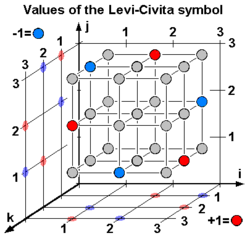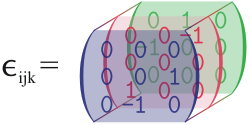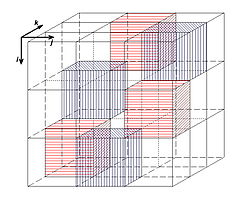# Levi-Civita symbol

Levi-Civita symbol

The Levi-Civita symbol, also called the permutation symbol, antisymmetric symbol, or alternating symbol, is a mathematical symbol used in particular in tensor calculus. It is named after the Italian mathematician and physicist Tullio Levi-Civita.

## DefinitionValues of the Levi-Civita-Symbol for a right-handed coordinate system.

In three dimensions, the Levi-Civita symbol is defined as follows:$\varepsilon_{ijk} = \begin{cases} +1 & \mbox{if } (i,j,k) \mbox{ is } (1,2,3), (3,1,2) \mbox{ or } (2,3,1), \\ -1 & \mbox{if } (i,j,k) \mbox{ is } (1,3,2), (3,2,1) \mbox{ or } (2,1,3), \\ 0 & \mbox{if }i=j \mbox{ or } j=k \mbox{ or } k=i \end{cases}$

i.e.$\varepsilon_{ijk}$ is 1 if (i, j, k) is an even permutation of (1,2,3), −1 if it is an odd permutation, and 0 if any index is repeated.

The formula for the three dimensional Levi-Civita symbol is:$\varepsilon_{ijk} = \frac{\left( j-i \right)\left( k-i \right)\left( k-j \right)}{2} = \frac{\left( i-j \right)\left( j-k \right)\left( k-i \right)}{2}$

The formula in four dimensions is:$\varepsilon_{ijkl} = \frac{\left( j-i \right)\left( k-i \right)\left( l-i \right)\left( k-j \right)\left( l-j \right)\left( l-k \right)}{12} = \frac{\left( i-j \right)\left( i-k \right)\left( i-l \right)\left( j-k \right)\left( j-l \right)\left( k-l \right)}{12}$Visualization of the Levi-Civita symbol as a 3×3×3 matrix.Corresponding visualization of the Levi-Civita-Symbol for a left-handed coordinate system. Empty cubes mean 0, red ones +1, and blue ones -1.

For example, in linear algebra, the determinant of a 3×3 matrix A can be written$\det A = \sum_{i=1}^3 \sum_{j=1}^3 \sum_{k=1}^3 \varepsilon_{ijk} a_{1i} a_{2j} a_{3k}$

(and similarly for a square matrix of general size, see below)

and the cross product of two vectors can be written as a determinant:$\mathbf{a \times b} = \begin{vmatrix} \mathbf{e_1} & \mathbf{e_2} & \mathbf{e_3} \\ a_1 & a_2 & a_3 \\ b_1 & b_2 & b_3 \\ \end{vmatrix} = \sum_{i=1}^3 \sum_{j=1}^3 \sum_{k=1}^3 \varepsilon_{ijk} \mathbf{e_i} a_j b_k$

or more simply:$\mathbf{a \times b} = \mathbf{c},\ c_i = \sum_{j=1}^3 \sum_{k=1}^3 \varepsilon_{ijk} a_j b_k.$

According to the Einstein notation, the summation symbols may be omitted.

The tensor whose components in an orthonormal basis are given by the Levi-Civita symbol (a tensor of covariant rank n) is sometimes called the permutation tensor. It is actually a pseudotensor because under an orthogonal transformation of jacobian determinant −1 (i.e., a rotation composed with a reflection), it acquires a minus sign. Because the Levi-Civita symbol is a pseudotensor, the result of taking a cross product is a pseudovector, not a vector.

Note that under a general coordinate change, the components of the permutation tensor get multiplied by the jacobian of the transformation matrix. This implies that in coordinate frames different from the one in which the tensor was defined, its components can differ from those of the Levi-Civita symbol by an overall factor. If the frame is orthonormal, the factor will be ±1 depending on whether the orientation of the frame is the same or not.

### Relation to Kronecker delta

The Levi-Civita symbol is related to the Kronecker delta. In three dimensions, the relationship is given by the following equations:$\varepsilon_{ijk}\varepsilon_{lmn} = \det \begin{bmatrix} \delta_{il} & \delta_{im}& \delta_{in}\\ \delta_{jl} & \delta_{jm}& \delta_{jn}\\ \delta_{kl} & \delta_{km}& \delta_{kn}\\ \end{bmatrix}$$= \delta_{il}\left( \delta_{jm}\delta_{kn} - \delta_{jn}\delta_{km}\right) - \delta_{im}\left( \delta_{jl}\delta_{kn} - \delta_{jn}\delta_{kl} \right) + \delta_{in} \left( \delta_{jl}\delta_{km} - \delta_{jm}\delta_{kl} \right) \,$$\sum_{i=1}^3 \varepsilon_{ijk}\varepsilon_{imn} = \delta_{jm}\delta_{kn} - \delta_{jn}\delta_{km}$ ("contracted epsilon identity")

In Einstein notation, the duplication of the i index implies the sum on i. The previous is then denoted:$\varepsilon_{ijk}\varepsilon_{imn} = \delta_{jm}\delta_{kn} - \delta_{jn}\delta_{km}\,.$$\sum_{i=1}^3 \sum_{j=1}^3 \varepsilon_{ijk}\varepsilon_{ijn} = 2\delta_{kn}$

### Generalization to n dimensions

The Levi-Civita symbol can be generalized to higher dimensions:$\varepsilon_{ijkl\dots} = \begin{cases} +1 & \mbox{if }(i,j,k,l,\dots) \mbox{ is an even permutation of } (1,2,3,4,\dots) \\ -1 & \mbox{if }(i,j,k,l,\dots) \mbox{ is an odd permutation of } (1,2,3,4,\dots) \\ 0 & \mbox{otherwise} \end{cases}$

Thus, it is the sign of the permutation in the case of a permutation, and zero otherwise.

The generalized formula is:$\varepsilon_{a_1 a_2 a_3 \ldots a_n} = \prod_{i=1}^{n-1} \left( \frac{1}{i!} \ \prod_{j=i+1}^n ( a_j-a_i ) \right) = \frac{\prod_{i

where n is the dimension (rank) and G(n) is the Barnes G-function.

For any n the property$\sum_{i,j,k,\dots=1}^n \varepsilon_{ijk\dots}\varepsilon_{ijk\dots} = n!$

follows from the facts that (a) every permutation is either even or odd, (b) (+1)2 = (-1)2 = 1, and (c) the permutations of any n-element set number exactly n!.

In index-free tensor notation, the Levi-Civita symbol is replaced by the concept of the Hodge dual.

In general, for n dimensions, one can write the product of two Levi-Civita symbols as:$\varepsilon_{i_1 i_2 \dots i_n} \varepsilon_{j_1 j_2 \dots j_n} = \det \begin{bmatrix} \delta_{i_1 j_1} & \delta_{i_1 j_2} & \dots & \delta_{i_1 j_n} \\ \delta_{i_2 j_1} & \delta_{i_2 j_2} & \dots & \delta_{i_2 j_n} \\ \vdots & \vdots & \ddots & \vdots \\ \delta_{i_n j_1} & \delta_{i_n j_2} & \dots & \delta_{i_n j_n} \\ \end{bmatrix}$.

## Properties

(in these examples, superscripts should be considered equivalent with subscripts)

1. In two dimensions, when all i,j,m,n are in {1,2},\begin{align}&\varepsilon_{ij} \varepsilon^{mn} = \delta^m_i \delta^n_j - \delta^n_i \delta^m_j \quad &&(1) \\&\varepsilon_{ij} \varepsilon^{in} = \delta^n_j &&(2) \\&\varepsilon_{ij} \varepsilon^{ij} = 2 &&(3)\end{align}

2. In three dimensions, when all i,j,k,m,n are in {1,2,3},\begin{align}&\varepsilon_{jmn} \varepsilon^{imn}=2\delta^i_j &&(4)\\&\varepsilon_{ijk} \varepsilon^{ijk}=6 &&(5)\\&\varepsilon_{ijk} \varepsilon^{imn}=\delta^{m}_j\delta^{n}_k - \delta^{n}_j\delta^{m}_k &&(6)\end{align}

3. In n dimensions, when all i1,...,in,j1,...,jn are in {1,...,n},:\begin{align}& \varepsilon_{i_1 \dots i_n} \varepsilon^{j_1 \dots j_n} = n! \delta^{j_1}_{[ i_1} \dots \delta^{j_n}_{i_n ]} &&(7)\\& \varepsilon_{i_1 \dots i_k~i_{k+1}\dots i_n} \varepsilon^{i_1 \dots i_k~j_{k+1}\dots j_n}= k!(n-k)!~\delta^{j_{k+1}}_{[ i_{k+1}} \dots \delta^{j_n}_{i_n ]} &&(8)\\& \varepsilon_{i_1 \dots i_n}\varepsilon^{i_1 \dots i_n} = n! &&(9)\end{align}

### Proofs

For equation 1, both sides are antisymmetric with respect of ij and mn. We therefore only need to consider the case$i\neq j$ and$m\neq n$. By substitution, we see that the equation holds for ε12ε12, i.e., for i = m = 1 and j = n = 2. (Both sides are then one). Since the equation is antisymmetric in ij and mn, any set of values for these can be reduced to the above case (which holds). The equation thus holds for all values of ij and mn. Using equation 1, we have for equation 2 εijεin =$\delta_i^i \delta_j^n - \delta^n_i \delta^i_j$

=$2 \delta_j^n - \delta^n_j$
=$\delta_j^n$.

Here we used the Einstein summation convention with i going from 1 to 2. Equation 3 follows similarly from equation 2. To establish equation 4, let us first observe that both sides vanish when$i\neq j$. Indeed, if$i\neq j$, then one can not choose m and n such that both permutation symbols on the left are nonzero. Then, with i = j fixed, there are only two ways to choose m and n from the remaining two indices. For any such indices, we have εjmnεimn = (εimn)2 = 1 (no summation), and the result follows. Property (5) follows since 3! = 6 and for any distinct indices i,j,k in {1,2,3}, we have εijkεijk = 1 (no summation).

## Examples

1. The determinant of an$n\times n$ matrix A = (aij) can be written as$\det A = \varepsilon_{i_1\cdots i_n} a_{1i_1} \cdots a_{ni_n},$

where each il should be summed over$1,\ldots, n.$

Equivalently, it may be written as$\det A = \frac{1}{n!} \varepsilon_{i_1\cdots i_n} \varepsilon_{j_1\cdots j_n} a_{i_1 j_1} \cdots a_{i_n j_n},$

where now each il and each jl should be summed over$1,\ldots, n$.

2. If A = (A1,A2,A3) and B = (B1,B2,B3) are vectors in R3 (represented in some right hand oriented orthonormal basis), then the ith component of their cross product equals$(A\times B)^i = \varepsilon^{ijk} A^j B^k.$

For instance, the first component of$A\times B$ is A2B3A3B2. From the above expression for the cross product, it is clear that$A\times B = -B\times A$. Further, if C = (C1,C2,C3) is a vector like A and B, then the triple scalar product equals$A\cdot(B\times C) = \varepsilon^{ijk} A^i B^j C^k.$

From this expression, it can be seen that the triple scalar product is antisymmetric when exchanging any adjacent arguments. For example,$A\cdot(B\times C)= -B\cdot(A\times C)$.

3. Suppose F = (F1,F2,F3) is a vector field defined on some open set of R3 with Cartesian coordinates x = (x1,x2,x3). Then the ith component of the curl of F equals$(\nabla \times F)^i(x) = \varepsilon^{ijk}\frac{\partial}{\partial x^j} F^k(x).$

## Notation

A shorthand notation for anti-symmetrization is denoted by a pair of square brackets. For example, in arbitrary dimensions, for a rank 2 covariant tensor M,$M_{[ab]} = \frac{1}{2!}(M_{ab} - M_{ba}) \,,$

and for a rank 3 covariant tensor T,$T_{[abc]} = \frac{1}{3!}(T_{abc}-T_{acb}+T_{bca}-T_{bac}+T_{cab}-T_{cba}) \,.$

In three dimensions, these are equivalent to$M_{[ab]} = \varepsilon_{abc} \, \frac{1}{2!} \, \varepsilon^{dec} M_{de} \,,$$T_{[abc]} = \varepsilon_{abc} \, \frac{1}{3!} \, \varepsilon^{def} T_{def} \,.$

While in four dimensions, these are equivalent to$M_{[ab]} = \frac{1}{2!} \, \varepsilon_{abcd} \, \frac{1}{2!} \, \varepsilon^{efcd} M_{ef} \,,$$T_{[abc]} = \varepsilon_{abcd} \, \frac{1}{3!} \, \varepsilon^{efgd} T_{efg} \,.$

More generally, in n dimensions$S_{[a_1 \dots a_i]} = \frac{1}{(n-i)!} \varepsilon_{a_1 \dots a_i~b_1 \dots b_{n-i}} \frac{1}{i!} \varepsilon^{c_1 \dots c_i~b_1 \dots b_{n-i}} S_{c_1 \dots c_i} \,.$

## Tensor density

In any arbitrary curvilinear coordinate system and even in the absence of a metric on the manifold, the Levi-Civita symbol as defined above may be considered to be a tensor density field in two different ways. It may be regarded as a contravariant tensor density of weight +1 or as a covariant tensor density of weight -1. In four dimensions,$\varepsilon^{\alpha \beta \gamma \delta} = \varepsilon_{\alpha \beta \gamma \delta} \,.$

Notice that the value, and in particular the sign, does not change.

### Ordinary tensor

In the presence of a metric tensor field, one may define an ordinary contravariant tensor field which agrees with the Levi-Civita symbol at each event whenever the coordinate system is such that the metric is orthonormal at that event. Similarly, one may also define an ordinary covariant tensor field which agrees with the Levi-Civita symbol at each event whenever the coordinate system is such that the metric is orthonormal at that event. These ordinary tensor fields should not be confused with each other, nor should they be confused with the tensor density fields mentioned above. One of these ordinary tensor fields may be converted to the other by raising or lowering the indices with the metric as is usual, but a minus sign is needed if the metric signature contains an odd number of negatives. For example, in Minkowski space (the four dimensional spacetime of special relativity)$E^{\alpha \beta \gamma \delta} = - g^{\alpha \zeta} g^{\beta \eta} g^{\gamma \theta} g^{\delta \iota} E_{\zeta \eta \theta \iota} \,.$

Notice the minus sign.

Wikimedia Foundation. 2010.

### Look at other dictionaries:

• Levi-Civita-Symbol — Das Levi Civita Symbol , auch Permutationssymbol, (ein wenig nachlässig) total antisymmetrischer Tensor oder Epsilon Tensor genannt, ist ein Symbol, das in der Physik bei der Vektor und Tensorrechnung nützlich ist. Es ist nach dem italienischen… …   Deutsch Wikipedia

• Levi-Cività-Symbol — Das Levi Civita Symbol , auch Permutationssymbol, (ein wenig nachlässig) total antisymmetrischer Tensor oder Epsilon Tensor genannt, ist ein Symbol, das in der Physik bei der Vektor und Tensorrechnung nützlich ist. Das Symbol bezeichnet die… …   Deutsch Wikipedia

• Levi-Cevita-Symbol — Das Levi Civita Symbol , auch Permutationssymbol, (ein wenig nachlässig) total antisymmetrischer Tensor oder Epsilon Tensor genannt, ist ein Symbol, das in der Physik bei der Vektor und Tensorrechnung nützlich ist. Das Symbol bezeichnet die… …   Deutsch Wikipedia

• Levi-Civita-Tensor — Das Levi Civita Symbol , auch Permutationssymbol, (ein wenig nachlässig) total antisymmetrischer Tensor oder Epsilon Tensor genannt, ist ein Symbol, das in der Physik bei der Vektor und Tensorrechnung nützlich ist. Das Symbol bezeichnet die… …   Deutsch Wikipedia

• Levi-Civita — Tullio Levi Civita (* 29. März 1873 in Padua; † 29. Dezember 1941 in Rom) war ein italienischer Mathematiker. Er war Schüler von Gregorio Ricci Curbastro und wurde 1898 Professor für Mechanik in Padua. 1918 ging er dann nach Rom. 1938 wurde er… …   Deutsch Wikipedia

• Levi-Civita — * Tullio Levi Civita, a Jewish Italian mathematician * Levi Civita (crater), a crater on the Moon * Levi Civita symbol, a mathematical symbol, also called permutation symbol or antisymmetric symbol …   Wikipedia

• Tullio Levi-Civita — Infobox Scientist name = Tullio Levi Civita box width = image width = caption = Tullio Levi Civita birth date = March 29, 1873 birth place = Padua death date = December 29, 1941 death place = residence = citizenship = nationality = Italian… …   Wikipedia

• 12473 Levi-Civita — Infobox Planet minorplanet = yes width = 25em bgcolour = #FFFFC0 apsis = name = Levi Civita symbol = caption = discovery = yes discovery ref = discoverer = P. G. Comba discovery site = Prescott discovered = February 10, 1997 designations = yes mp …   Wikipedia

• Levi (disambiguation) — Levi was, according to the Book of Genesis, the founder of the Israelite Tribe of Levi.Levi may also refer to: * The Laevi, or Levi, a Ligurian people in Gallia Transpadana * Levi II (fl. 3rd century), one of the amoraim * Levi (New Testament),… …   Wikipedia

• 't Hooft symbol — The t Hooft η symbol is a symbol which allows one to express the generators of the SU(2) Lie algebra in terms of the generators of Lorentz algebra. The symbol is a blend between the Kronecker delta and the Levi Civita symbol. It was introduced by …   Wikipedia